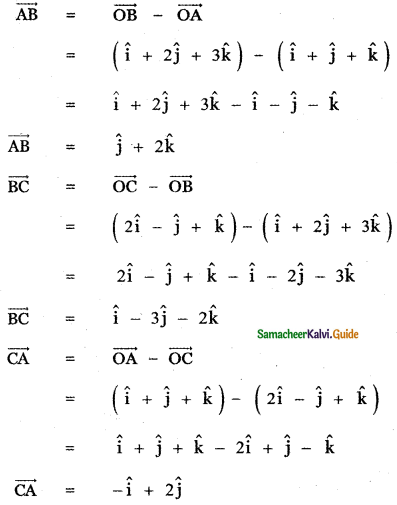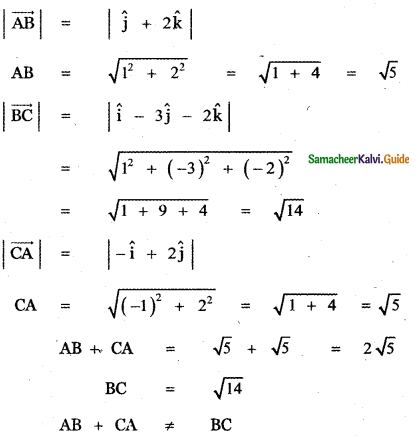Tamilnadu State Board New Syllabus Samacheer Kalvi 11th Maths Guide Pdf Chapter 8 Vector Algebra – I Ex 8.2 Text Book Back Questions and Answers, Notes.

## Tamilnadu Samacheer Kalvi 11th Maths Solutions Chapter 8 Vector Algebra – I Ex 8.2Question 1.
Verify whether the following ratios are direction cosines of some vector or not.
(i) $$\frac{1}{5}, \frac{3}{5}, \frac{4}{5}$$
(ii) $$\frac{1}{\sqrt{2}}, \frac{1}{2}, \frac{1}{2}$$
(iii) $$\frac{4}{3}, 0, \frac{3}{4}$$
(i) $$\frac{1}{5}, \frac{3}{5}, \frac{4}{5}$$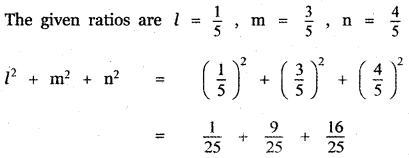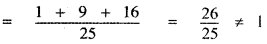[If l, m, n are direction cosines of a vector then l2 + m2 + n2 = 1]
∴ The given ratio $$\frac{1}{5}, \quad \frac{3}{5}, \quad \frac{4}{5}$$ do not form the direction cosines of a vector.

(ii) $$\frac{1}{\sqrt{2}}, \frac{1}{2}, \frac{1}{2}$$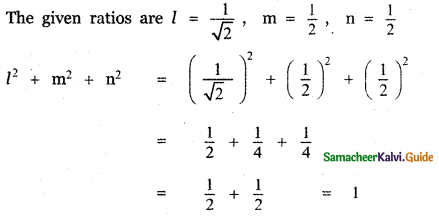[If l, m, n are direction cosines of a vector then l2 + m2 + n2 = 1]
∴ The given ratio form the direction cosines of a vector.

(iii) $$\frac{4}{3}, 0, \frac{3}{4}$$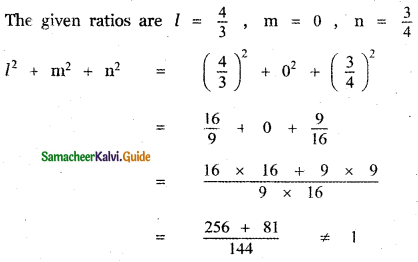[If l, m, n are direction cosines of a vector then l2 + m2 + n2 = 1]
∴ The given ratio do not form the direction cosines of a vector.Question 2.
Find the direction cosines of a vector whose direction ratios are
(i) 1, 2, 3
(ii) 3, -1 , 3
(iii) 0, 0, 7
(i) 1, 2, 3
The given direction ratios are a = 1, b = 2 , c = 3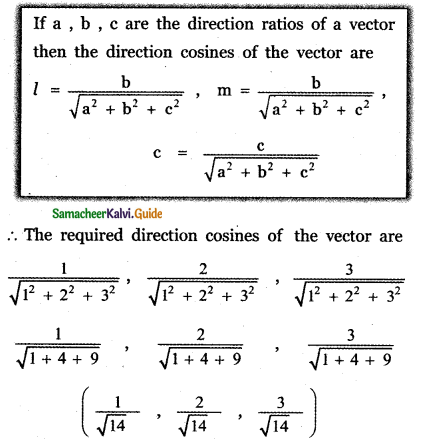(ii) 3, – 1, 3
The given direction ratios are a = 3, b = – 1 , c = 3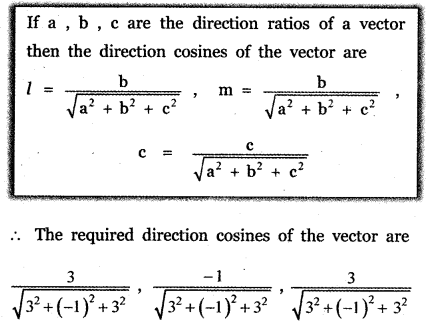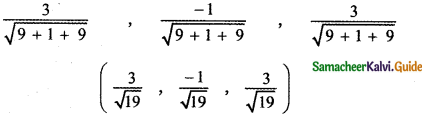(iii) 0, 0, 7
The given direction ratios are a = 0, b = 0, c = 7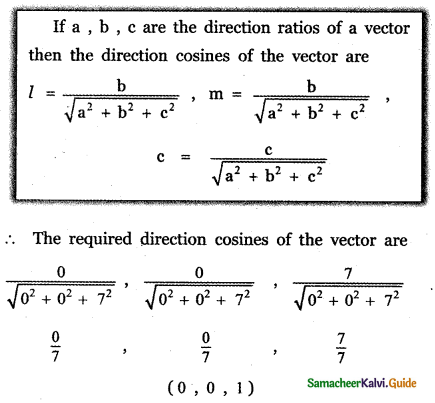Question 3.
Find the direction cosines and direction ratios for the following vectors.
(i) 3î – 4ĵ + 8k̂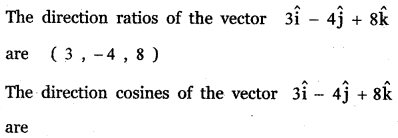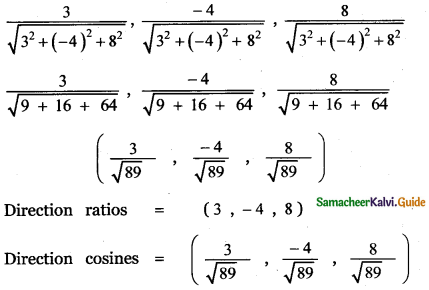(ii) 3î + ĵ + k̂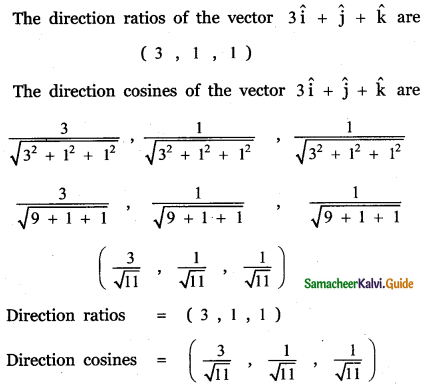(iii) ĵ
ĵ = 0î + ĵ + 0k̂
The direction ratios of the vector ĵ are (0, 1, 0)
The direction cosines of the vector ĵ are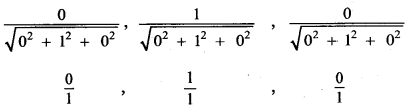(0, 1, 0)
Direction ratios = (0, 1, 0)
Direction cosines = (0, 1, 0)

(iv) 5î – 3ĵ – 48k̂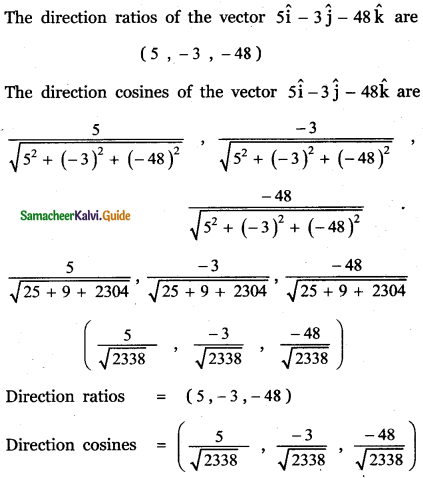(v) 3î – 3k̂ + 4ĵ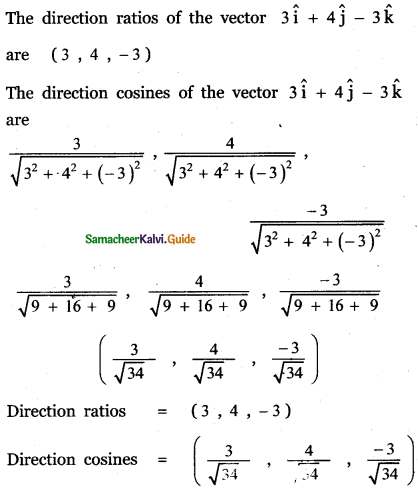(vi) î – k̂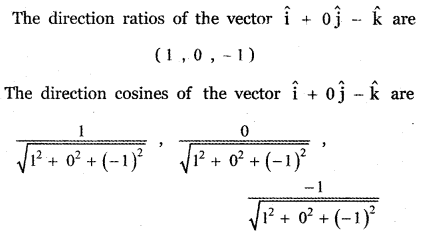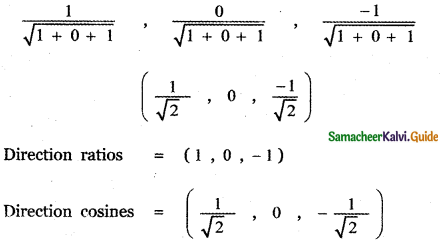Question 4.
A triangle is formed by joining the points (1, 0, 0), (0, 1, 0) and (0, 0, 1). Find the direction cosines of the medians.
Let ABC be the triangle and D, E, F is the midpoints of the sides BC, CA, AB respectively. Then AD, BE, CF are the medians of ∆ ABC.
Given that the vertices of the triangle are A (1, 0, 0) , B (0, 1, 0 ) and C (0, 0, 1).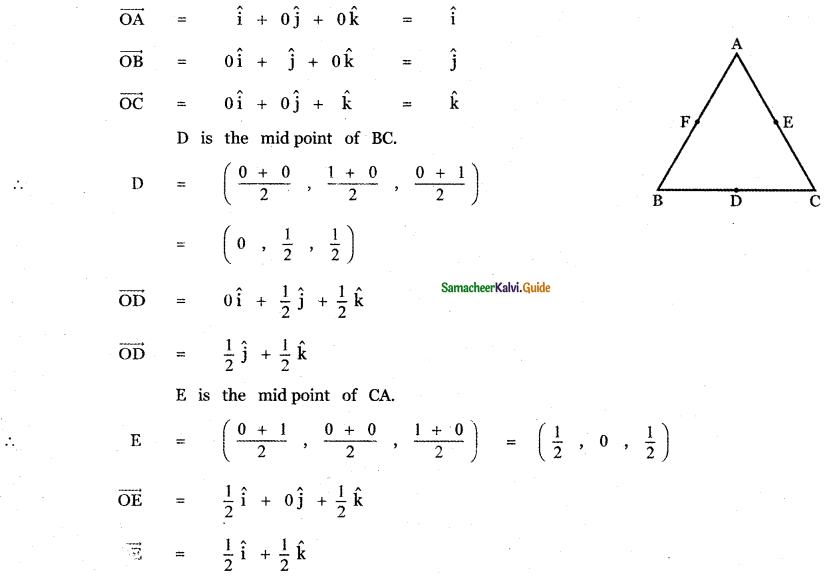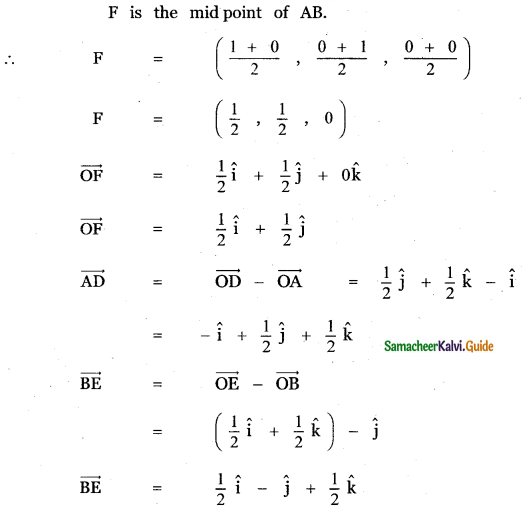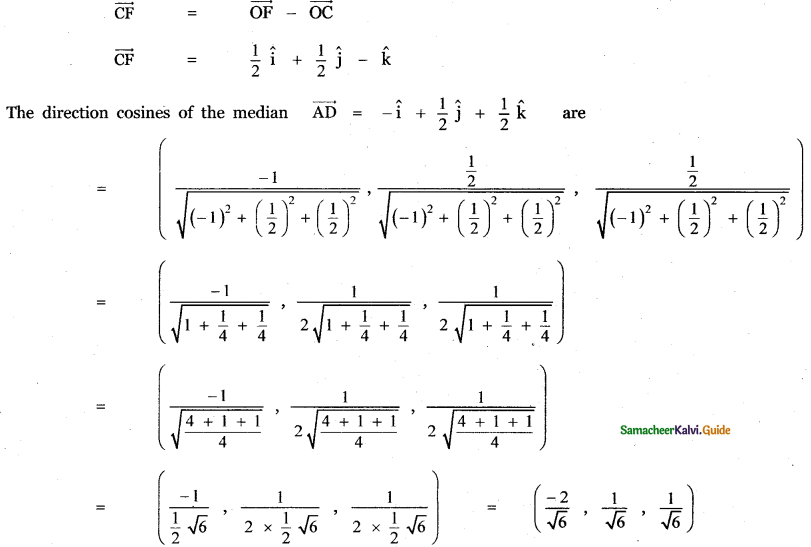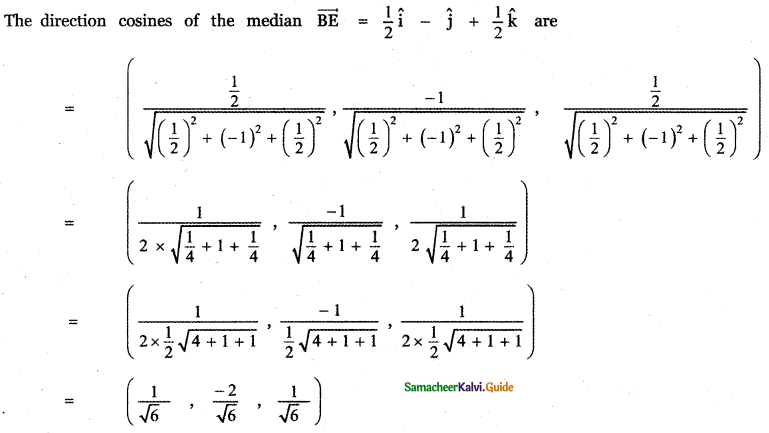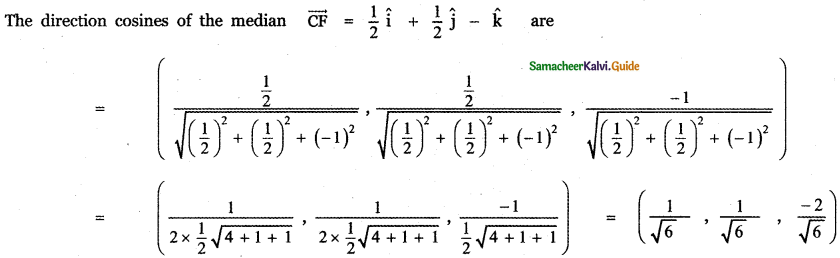Question 5.
If $$\frac{1}{2}, \frac{1}{\sqrt{2}}$$, a are the direction cosines of some vector, then find a.
Given $$\frac{1}{2}, \frac{1}{\sqrt{2}}$$, a are the direction cosines of some vector, then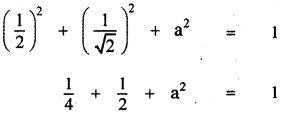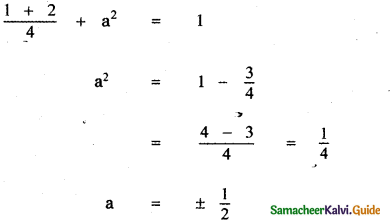[If l, m, n are direction cosines of a vector then l2 + m2 + n2 = 1]

Question 6.
If (a, a + b , a + b + c)is one set of direction ratios of the line joining (1, 0, 0) and (0, 1, 0), then find a set of values of a, b, c.
Let A be the point (1, 0, 0) and B be the point (0, 1, 0) (i.e.,) $$\overrightarrow{\mathrm{OA}}=\hat{i}$$ and $$\overrightarrow{\mathrm{OB}}=\hat{j}$$
Then $$\overrightarrow{\mathrm{AB}}=\overrightarrow{\mathrm{OB}}-\overrightarrow{\mathrm{OA}}=\hat{j}-\hat{i}=-\hat{i}+\hat{j}$$
= (-1, 1, 0)
= (a, a + b, a + b + c)
⇒ a = -1, a + b = 1 and a + b + c = 0
Now a = -1 ⇒ -1 + b = 1 ;a + b + c = 0
⇒ b = 2; -1 + 2 + c = 0 ⇒ c + 1 = 0
⇒ c = -1
∴ a = -1; b = 2; c = -1.
Note: If we taken $$\overrightarrow{\mathrm{BA}}$$ then we get a = 1, b = -2 and c = 1.Question 7.
Show that the vectors 2î – ĵ + k̂ , 3î – 4ĵ – 4k̂, î – 3ĵ – 5k̂ form a right angled triangle.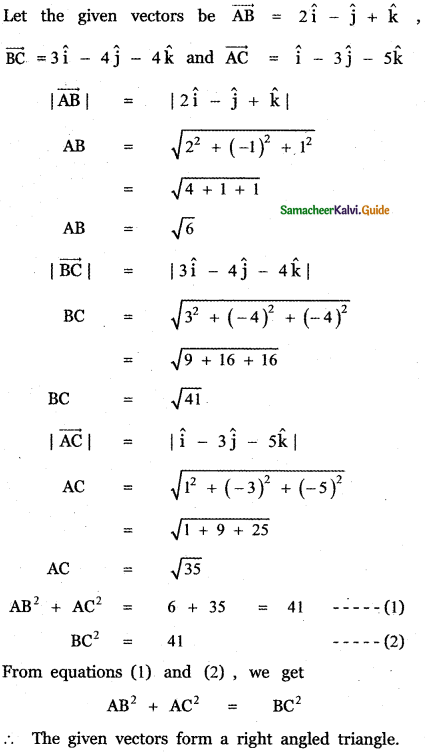Question 8.
Find the value of λ for which the vector $$\vec{a}$$ = 3î + 2ĵ + 9k̂ and $$\vec{b}$$ = î + λĵ + 3k̂ are parallel.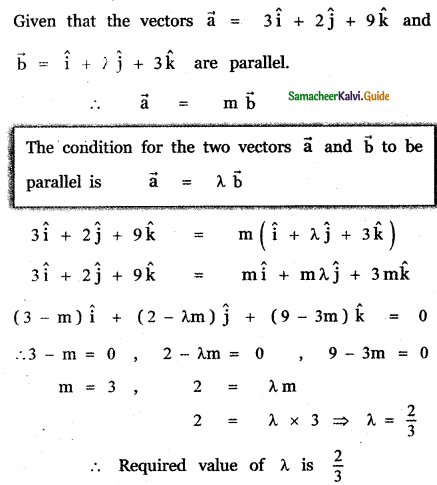Question 9.
Show that the following vectors are coplanar
(i) î – 2ĵ + 3k̂, – 2î + 3ĵ – 4k̂, – ĵ + 2k̂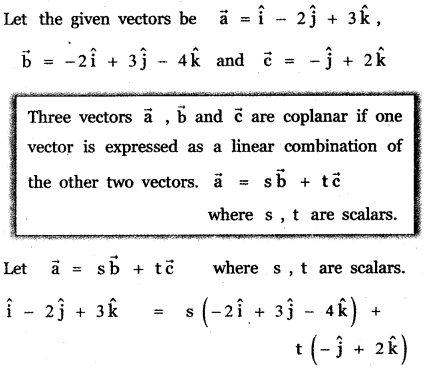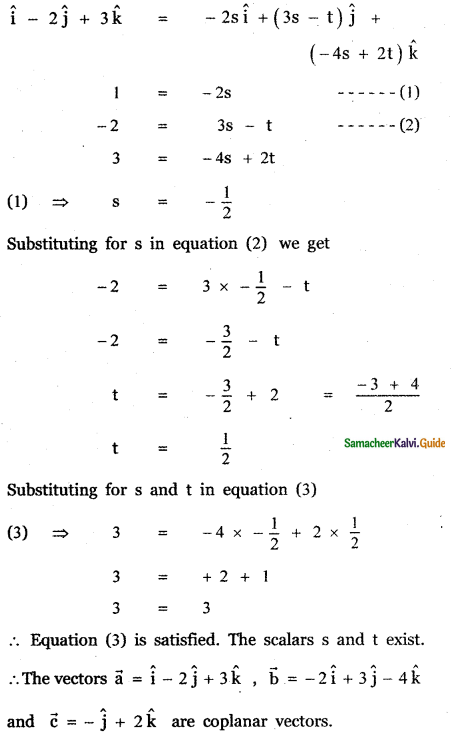(ii) 5î + 6ĵ + 7k̂, 7î – 8ĵ + 9k̂, 3î + 20ĵ + 5k̂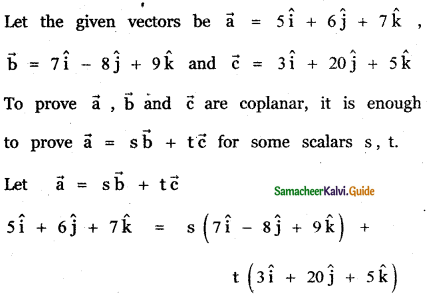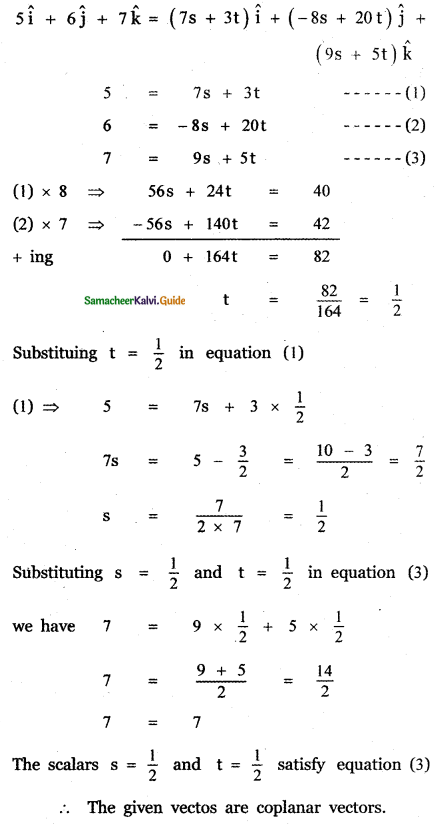Question 10.
Show that the points whose position vectors 4î + 5ĵ + k̂, – ĵ – k̂, 3î + 9ĵ + 4k̂ and -4î + 4ĵ + 4k̂ are coplanar.
Let the given position vectors of the points A, B, C, D be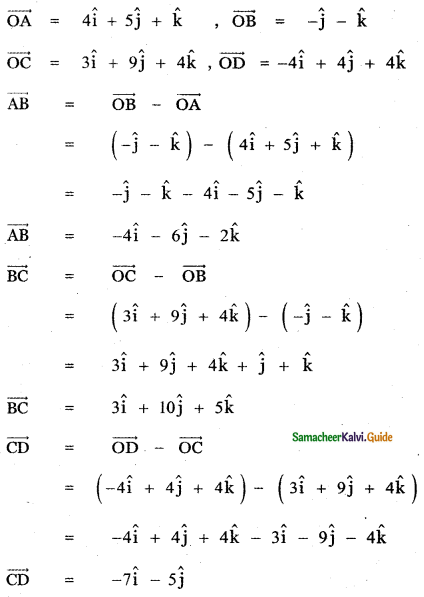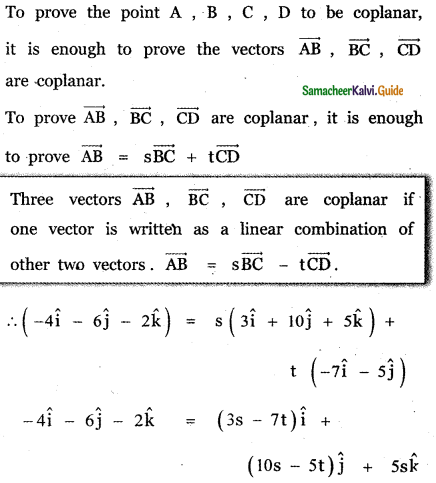Equating the like terms on both sides
-4 = 3s – 7t ………… (1)
-6 = 10s – 5t ……….. (2)
-2 = 5s …………. (3)
(3) ⇒ s = $$-\frac{2}{5}$$
Substituting in equation (2) , we have
-6 = 10 × $$-\frac{2}{5}$$ – 5t
-6 = -4 – 5t
-6 + 4 = -5t
⇒ -5t = -2
⇒ t = $$\frac{2}{5}$$
Substituting for s and t in equation (1), we haveQuestion 11.
If $$\vec{a}$$ = 2î + 3ĵ – 4k̂ , $$\vec{b}$$ = 3î – 4ĵ – 5k̂ and $$\vec{c}$$ = – 3î + 2ĵ + 3k̂ , find the magnitude and direction cosines of
(i) $$\vec{a}$$ + $$\vec{b}$$ + $$\vec{c}$$
(ii) 3$$\vec{a}$$ – 2$$\vec{b}$$ + 5$$\vec{c}$$
The given vectors are $$\vec{a}$$ = 2î + 3ĵ – 4k̂ , $$\vec{b}$$ = 3î – 4ĵ – 5k̂ $$\vec{c}$$ = – 3î + 2ĵ + 3k̂
(i) $$\vec{a}$$ + $$\vec{b}$$ + $$\vec{c}$$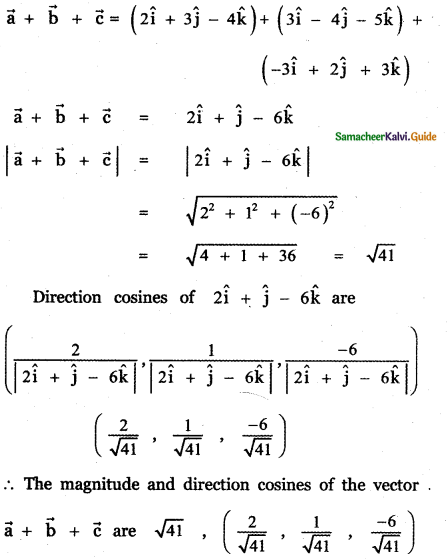(ii) 3$$\vec{a}$$ – 2$$\vec{b}$$ + 5$$\vec{c}$$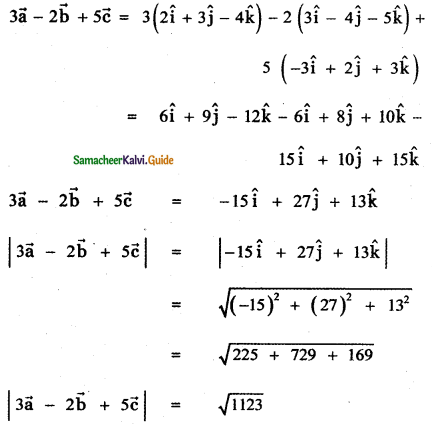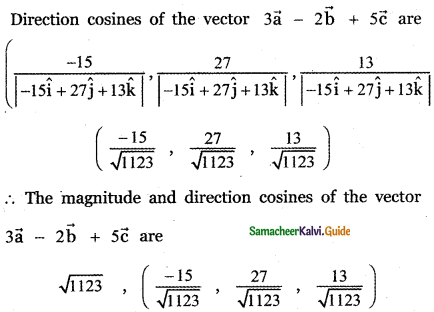Question 12.
The position vectors of the vertices of a triangle are î + 2ĵ + 3k̂ ; 3î – 4ĵ + 5k̂ and – 2î + 3ĵ – 7k̂. Find the perimeter of the triangle.
Let A, B, C be the vertices of a triangle ABC.
Given the position vectors of the vertices A, B, C are given by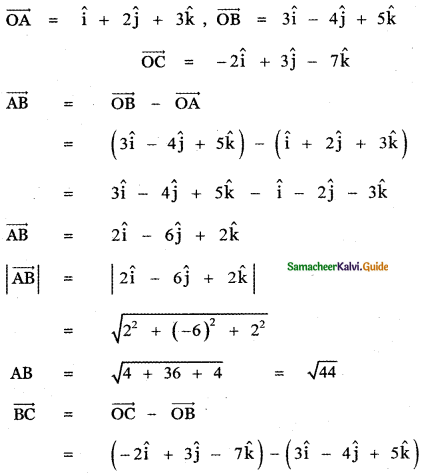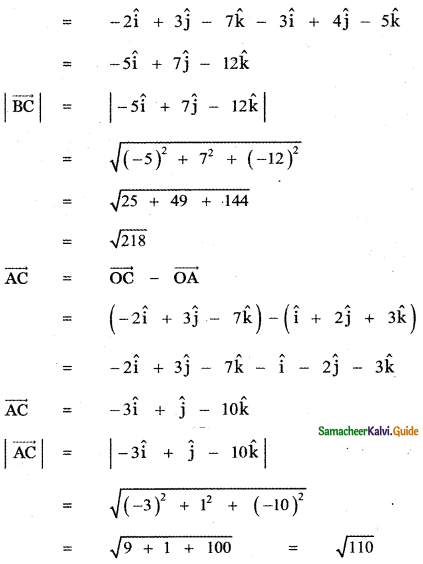∴ The required perimeter of the triangle ABC is given by AB + BC + AC = $$\sqrt{44}+\sqrt{218}+\sqrt{110}$$Question 13.
Find the unit vector parallel to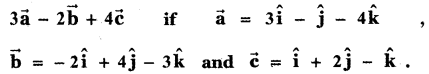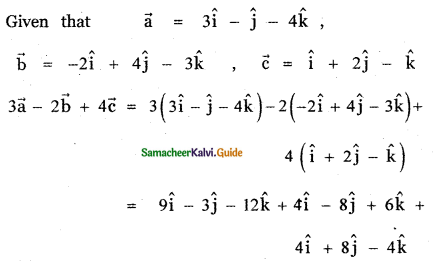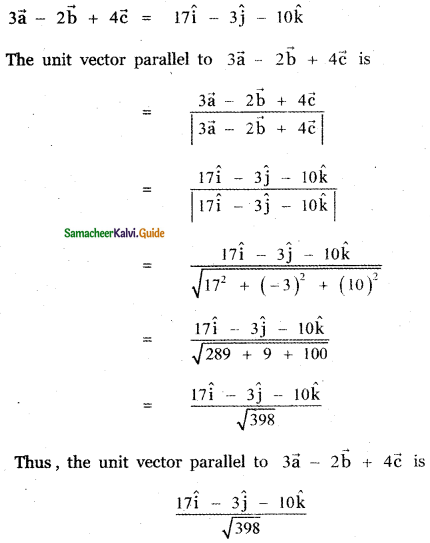Question 14.
The position vectors $$\vec{a}$$, $$\vec{b}$$, $$\vec{c}$$ of three points satisfy the relation 2 $$\vec{a}$$ + 7$$\vec{b}$$ + 5$$\vec{c}$$ = $$\vec{0}$$. Are these points collinear?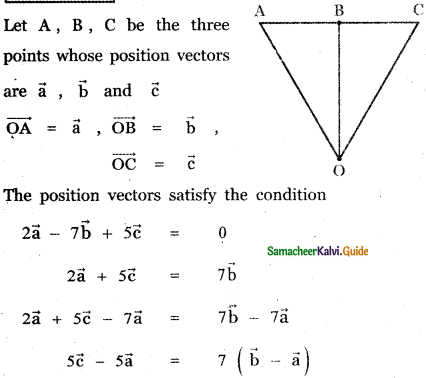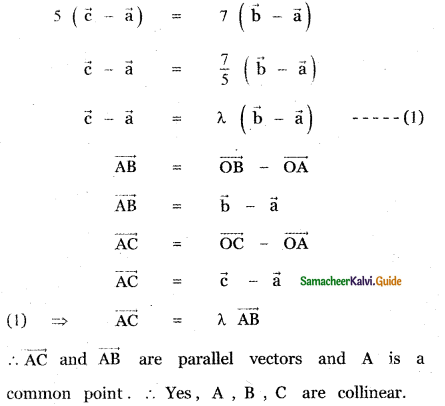Question 15.
The position vector of the points P, Q, R, S are î + ĵ + k̂, 2î + 5ĵ, 3î + 2ĵ – 3k̂ and î – 6ĵ – k̂ respectively. Prove that the line and PQ and RS are parallel.
Given that the position vector of the given points P, Q, R, S are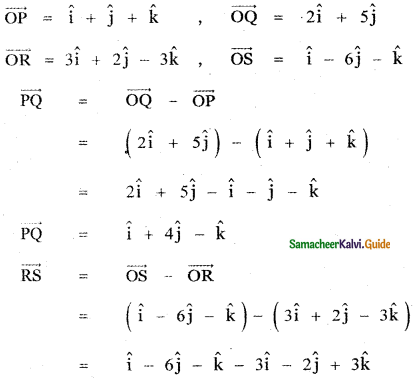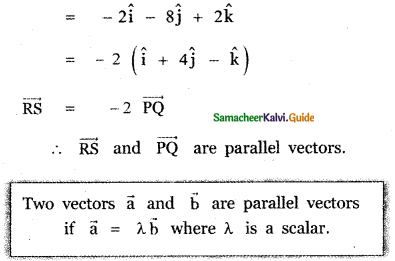Question 16.
Find the value or values of m for which m (î + ĵ + k̂) is a unit vector.
The given vector is m (î + ĵ + k̂)
Given that it is a unit vector.
∴ |m (i + j + k)| = 1
|m (i + j + k)| = 1
m (± $$\sqrt{1^{2}+1^{2}+1^{2}}$$) = 1
m = ± $$\frac{1}{\sqrt{3}}$$Question 17.
Show that points A (1, 1, 1), B (1, 2, 3), and C (2, -1, 1 ) are vertices of an isosceles triangle.
Position vector of A is $$\overrightarrow{\mathrm{OA}}$$ = î + ĵ + k̂
Position vector of B is $$\overrightarrow{\mathrm{OB}}$$ = î + 2ĵ + 3k̂
Position vector of C is $$\overrightarrow{\mathrm{OC}}$$ = 2î – ĵ + k̂